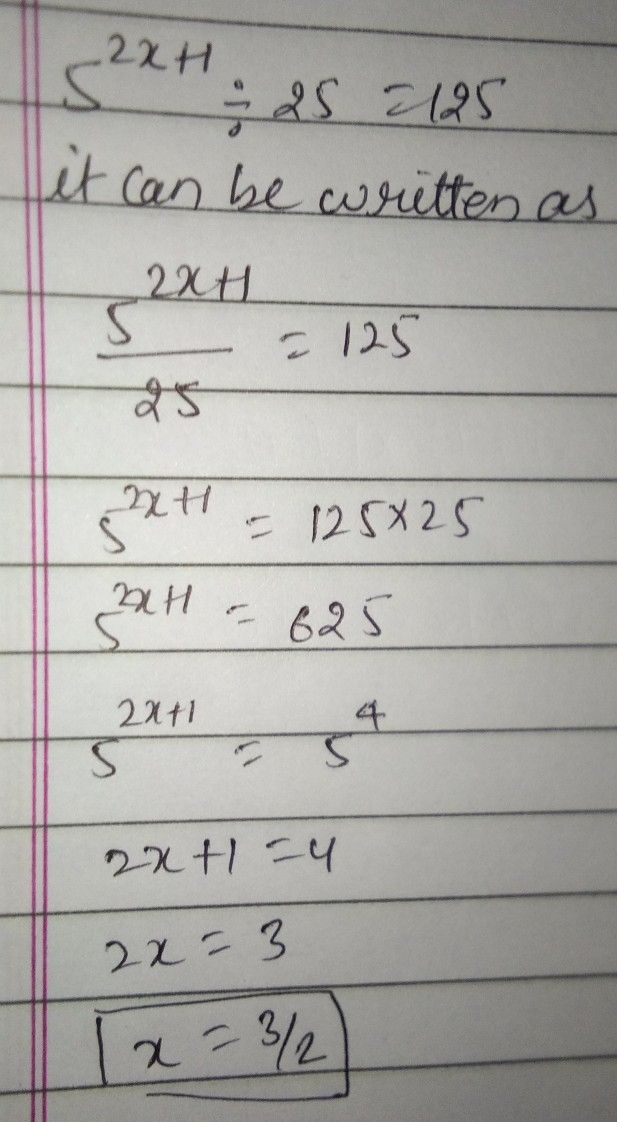Symbol
Problem$12$ By what number should $\bar{3}$ be div $18.$ $1f5^{2x+1}+25=125$ find the value of $x$
7th-9th grade
Other
SolutionQanda teacher - fairabhope you got it
any doubt then ask me